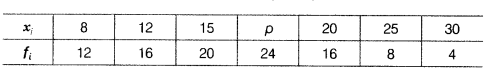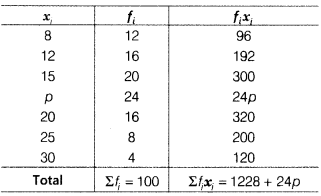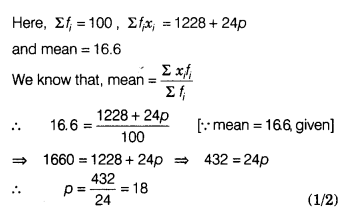# Find the value of p for the following frequency distribution, whose mean is 16.6

(i) Find the value of p for the following frequency distribution, whose mean is 16.6.Consider, the following table